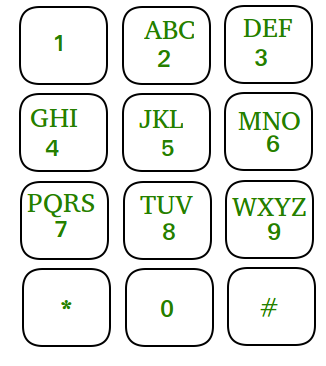# Print all n digit patterns formed by mobile Keypad

Given a number n. we need to print all N digit pattern formed by mobile Keypad.
Note: we can move up, down, left, right from any key of mobile keypad and every pattern contain unique key.Examples:

```
Input :   N = 3
Output :  all 3 digit Pattern are :
123, 125, 145, 147
236, 214, 258, 256, 254
321, 325, 369, 365
412, 456, 452, 458, 478
and so on ..
```

## Recommended: Please try your approach on {IDE} first, before moving on to the solution.

idea of this solution is based on the DFS. We pick all keypad key as a starting digit for N_digit number one by one, after that we are try to generate all N digit pattern formed by this key( Using DFS because we can only move either up, left, right or down from that key).

``` PrintPattern Function (DFS Function)
.... make current key as visited
visited[x][y] = true;
... Print pattern if size of Pattern == N
__DFS_function

```

Below c++ implementation of above idea

 `// C++ program to print all n digit patterns ` `// formed by mobile keypad. ` `#include ` `using` `namespace` `std; ` ` `  `// A function to check if a given cell (row, col) ` `// can be included in DFS ` `bool` `isSafe(``int` `x, ``int` `y, ``bool` `Visited[]) ` `{ ` `    ``// row number is in range, column number  ` `    ``// is in range and not yet visited ` `    ``return` `(x >= 0 && x < 4 && y >= 0 &&  ` `            ``y < 3 && !Visited[x][y]); ` `} ` ` `  `// A utility function to do DFS for a mobile Keypad ` `// matrix. It only considers the 4 neighbors as ` `// adjacent vertice and print pattern of size n ` `void` `DFS(``bool` `visited[], ``int` `Keypad[], ``int` `n, ` `         ``string pattern, ``int` `x, ``int` `y) ` `{ ` ` `  `    ``// add current number to string ` `    ``pattern.push_back((Keypad[x][y] + ``'0'``)); ` ` `  `    ``// print pattern ` `    ``if` `(pattern.size() == n) { ` `        ``cout << pattern << ``" "``; ` `        ``return``; ` `    ``} ` ` `  `    ``// These arrays are used to get row and  ` `    ``// column ` `    ``// numbers of 4 neighbours of a given cell ` `    ``static` `int` `row[] = { 0, 1, 0, -1 }; ` `    ``static` `int` `col[] = { 1, 0, -1, 0 }; ` ` `  `    ``// Mark this cell as visited ` `    ``visited[x][y] = ``true``; ` ` `  `    ``// Recur for all connected neighbours ` `    ``for` `(``int` `k = 0; k < 4; k++) ` `        ``if` `(isSafe(x + row[k], y + col[k], visited) ` `            ``&& Keypad[x + row[k]][y + col[k]] != -1) ` `            ``DFS(visited, Keypad, n, pattern, ` `                       ``x + row[k], y + col[k]); ` ` `  `    ``// unvisited ` `    ``visited[x][y] = ``false``; ` `    ``pattern.pop_back(); ` `} ` ` `  `void` `patternOfSizeK(``int` `Keypad[], ``int` `n) ` `{ ` `    ``// Make a bool array to mark visited cells. ` `    ``// Initially all cells are unvisited ` `    ``bool` `visited; ` `    ``memset``(visited, ``false``, ``sizeof``(visited)); ` ` `  `    ``// try to generate all pattern of size n ` `    ``// by making every key of keypad as ` `    ``// starting char of pattern ` `    ``for` `(``int` `i = 0; i < 4; i++) ` `        ``for` `(``int` `j = 0; j < 3; j++) ` `            ``if` `(Keypad[i][j] != -1) ` `                ``DFS(visited, Keypad, n, ``""``, i, j); ` `} ` ` `  `// Drive program to test above function. ` `int` `main() ` `{ ` `    ``int` `Keypad = { { 1, 2, 3 }, ` `                         ``{ 4, 5, 6 }, ` `                         ``{ 7, 8, 9 }, ` `                         ``{ -1, 0, -1 } }; ` `    ``int` `n = 3; ` `    ``patternOfSizeK(Keypad, n); ` `    ``return` `0; ` `} `

Output:

```123 125 145 147 236 256 258 254 214 369 365 325 321
456 458 452 478 412 569 563 589 580 587 547 541 523 521
698 658 654 652 632 789 780 785 745 741 896 874 856 854 852
980 987 985 965 963 089 087 085
```

Attention reader! Don’t stop learning now. Get hold of all the important DSA concepts with the DSA Self Paced Course at a student-friendly price and become industry ready.

My Personal Notes arrow_drop_upCheck out this Author's contributed articles.

If you like GeeksforGeeks and would like to contribute, you can also write an article using contribute.geeksforgeeks.org or mail your article to contribute@geeksforgeeks.org. See your article appearing on the GeeksforGeeks main page and help other Geeks.

Please Improve this article if you find anything incorrect by clicking on the "Improve Article" button below.

Article Tags :
Practice Tags :

Be the First to upvote.

Please write to us at contribute@geeksforgeeks.org to report any issue with the above content.